## Based on the above information, answer the following questions: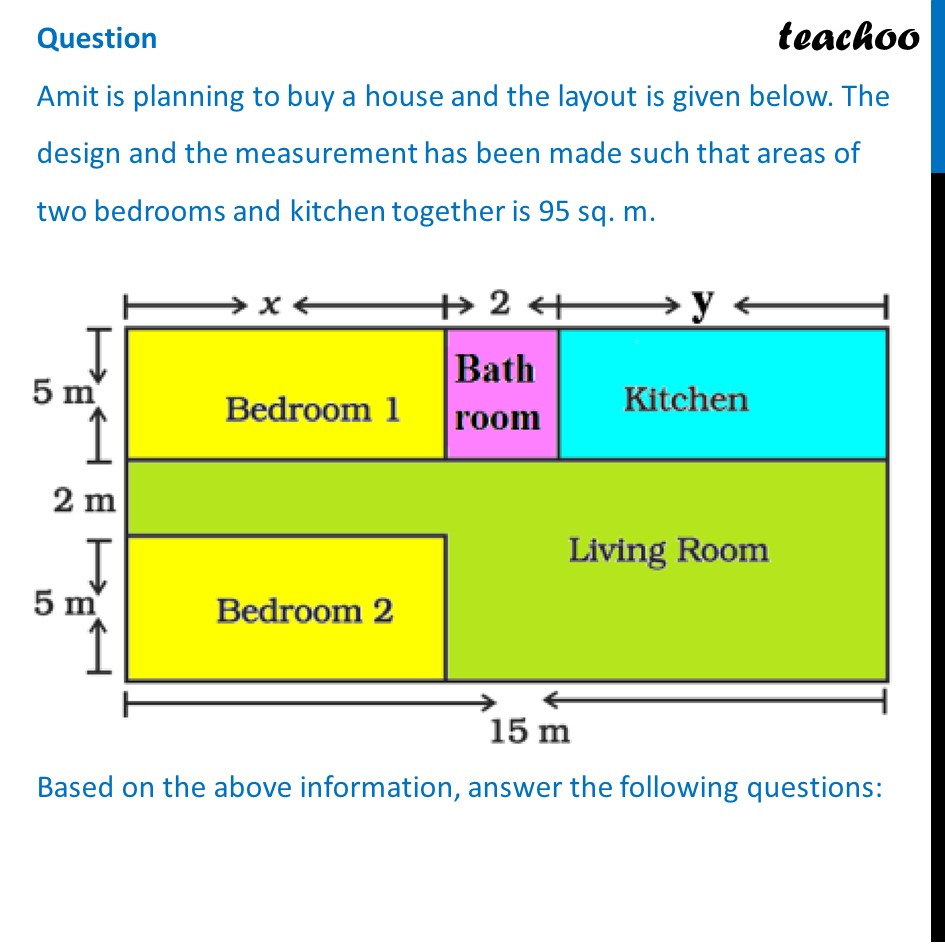## Form the pair of linear equations in two variables from this situation.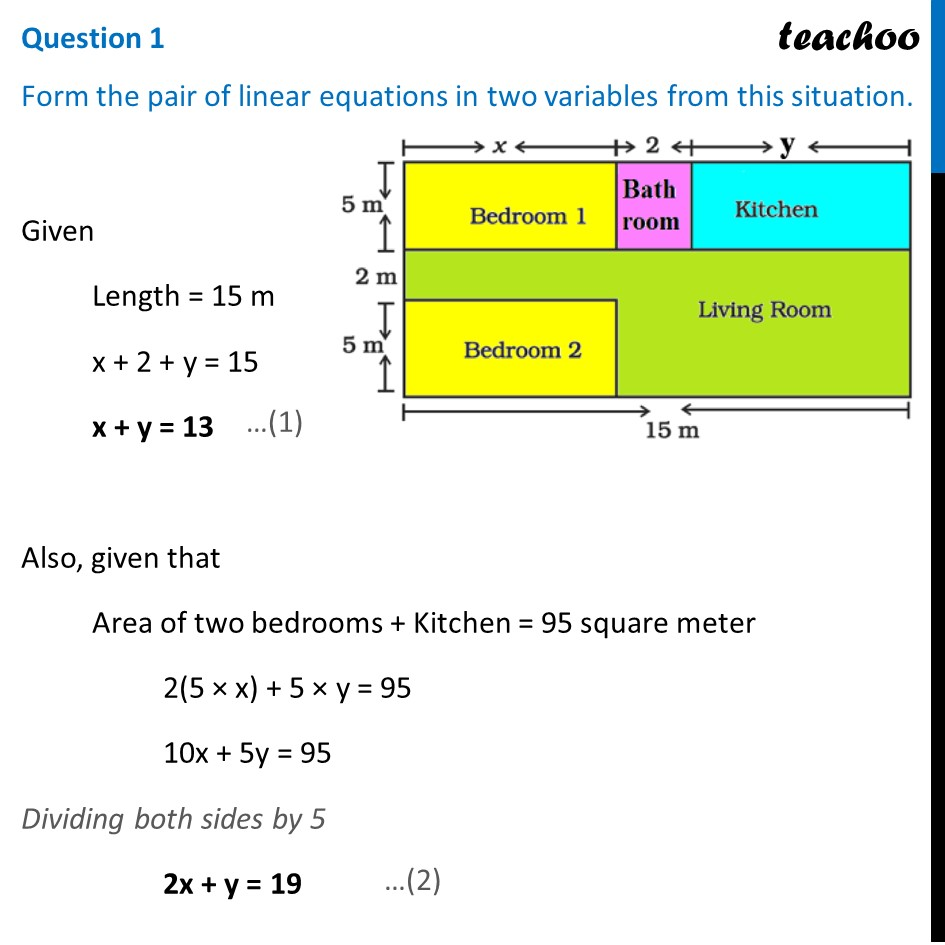## Find the length of the outer boundary of the layout.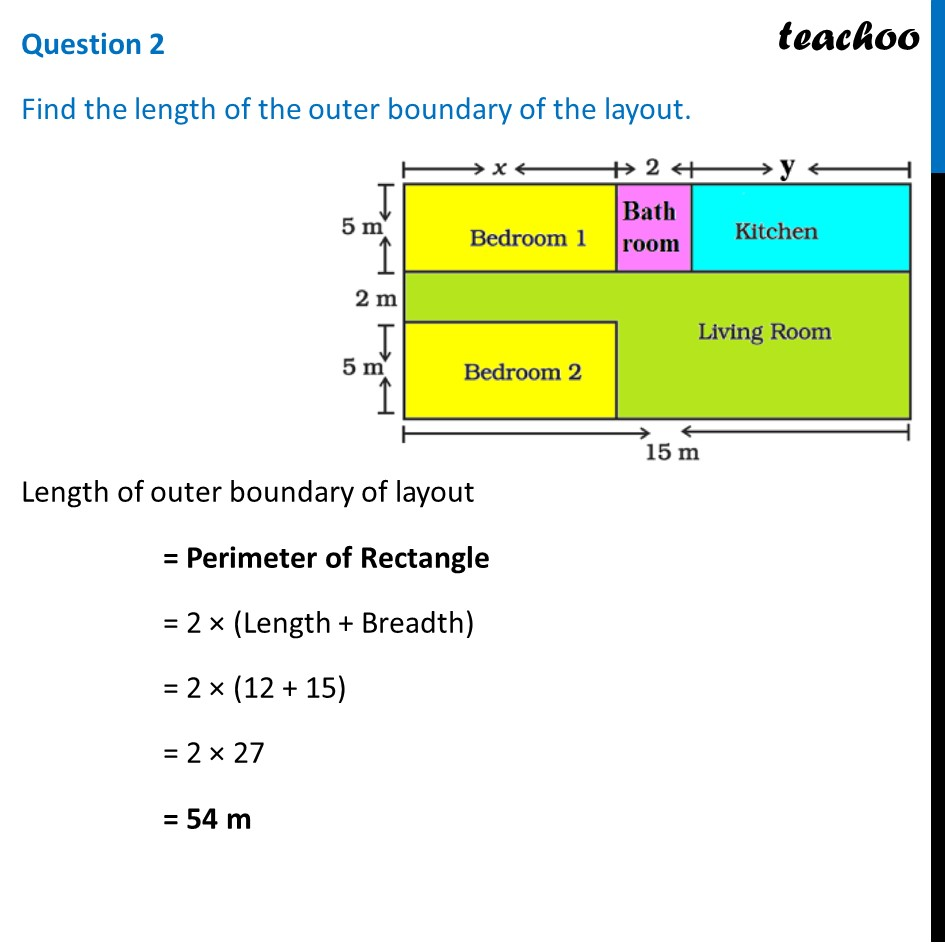## Find the area of each bedroom and kitchen in the layout.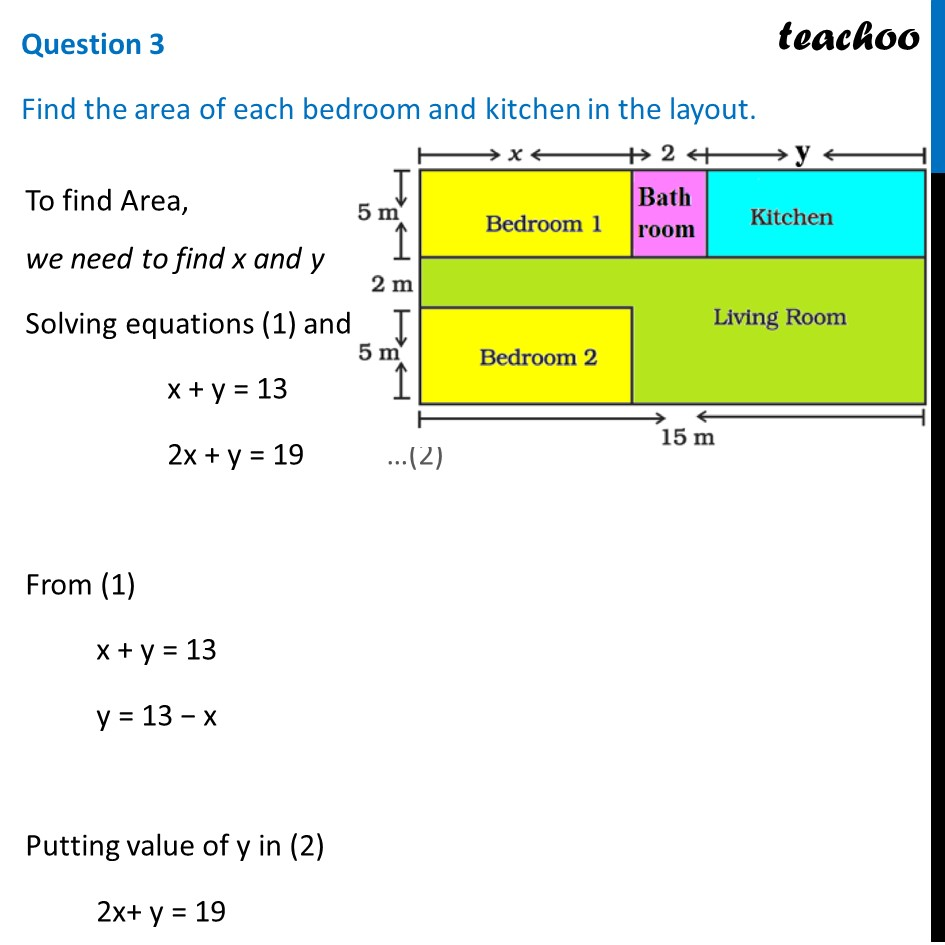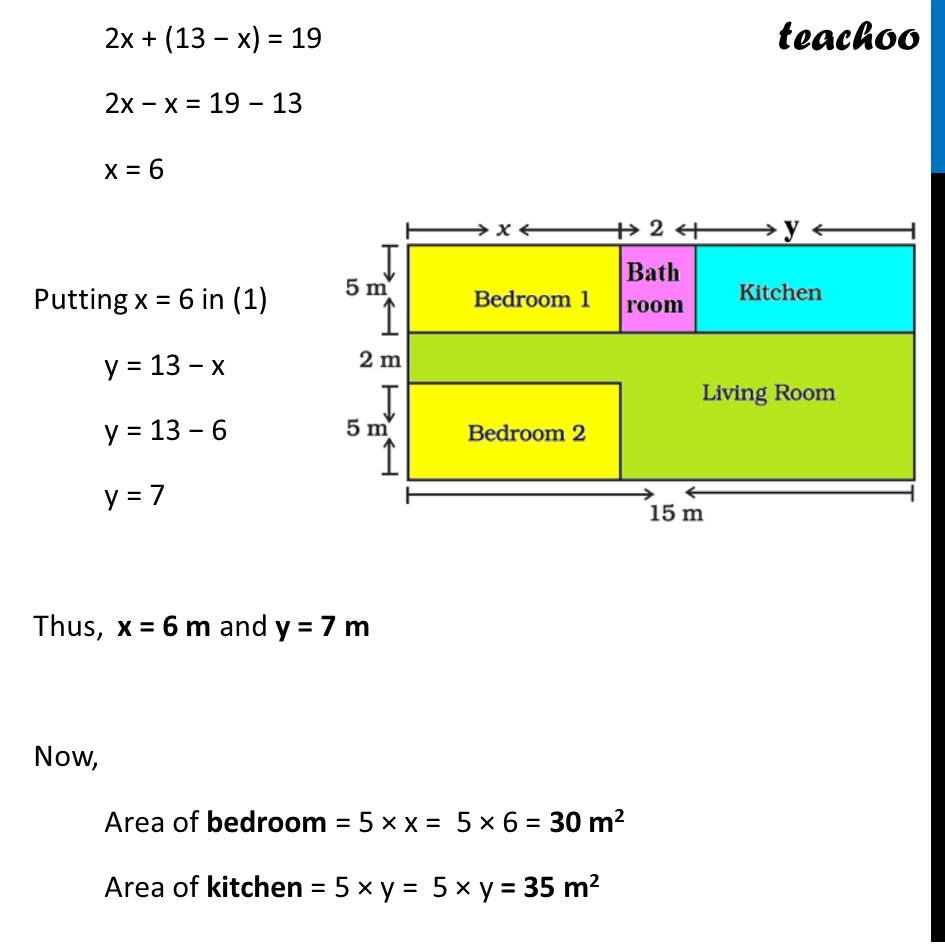## Find the area of living room in the layout.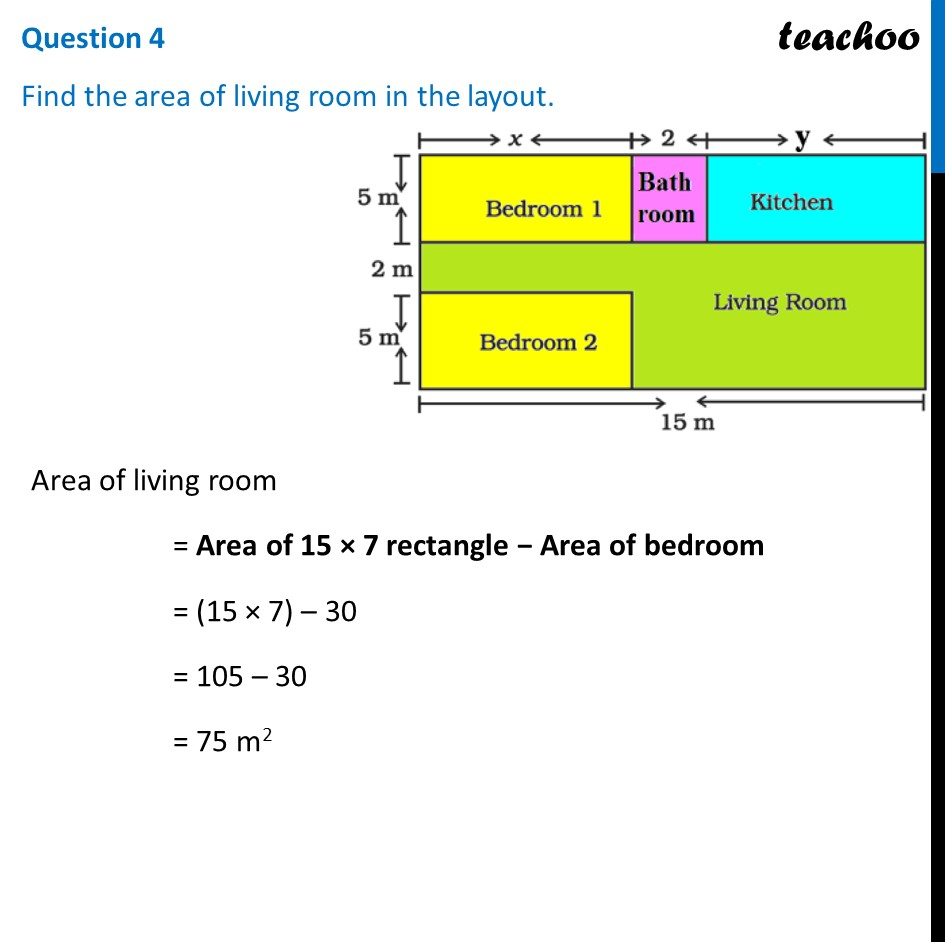##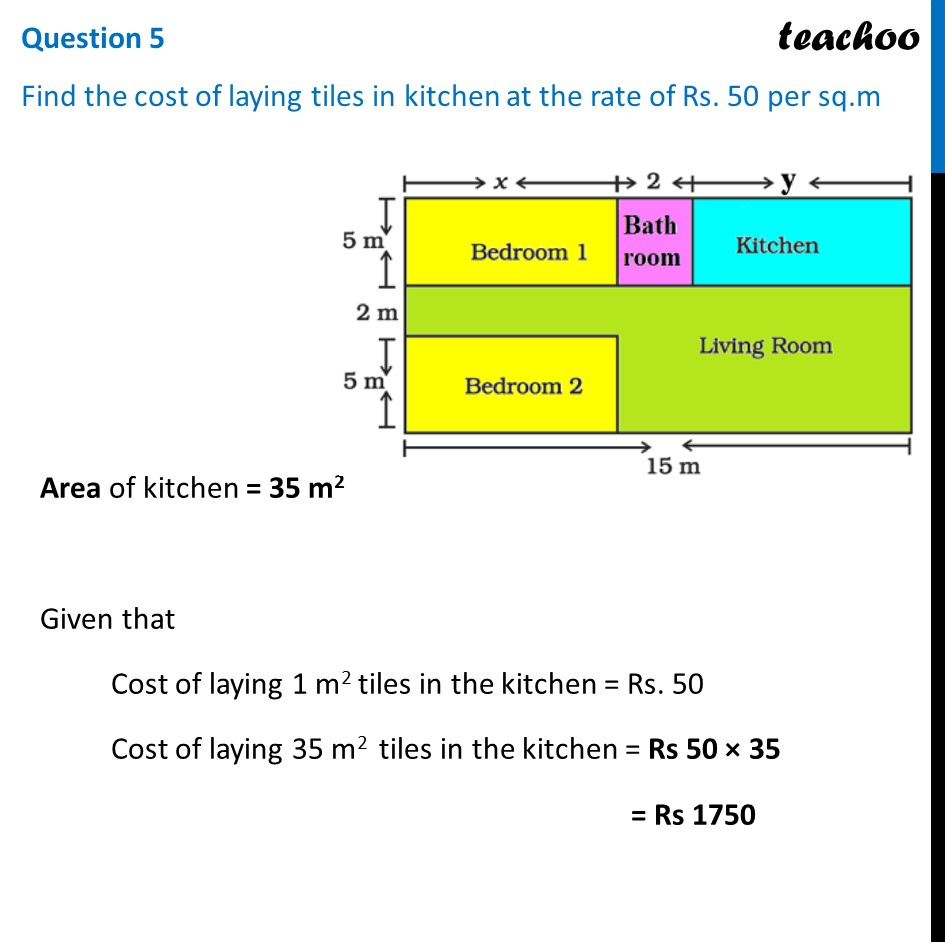1. Chapter 3 Class 10 Pair of Linear Equations in Two Variables
2. Serial order wise
3. Case Based Questions (MCQ)

Transcript

Question Amit is planning to buy a house and the layout is given below. The design and the measurement has been made such that areas of two bedrooms and kitchen together is 95 sq. m. Question 1 Form the pair of linear equations in two variables from this situation. Given Length = 15 m x + 2 + y = 15 x + y = 13 Also, given that Area of two bedrooms + Kitchen = 95 square meter 2(5 × x) + 5 × y = 95 10x + 5y = 95 Dividing both sides by 5 2x + y = 19 Question 2 Find the length of the outer boundary of the layout. Length of outer boundary of layout = Perimeter of Rectangle = 2 × (Length + Breadth) = 2 × (12 + 15) = 2 × 27 = 54 m Question 3 Find the area of each bedroom and kitchen in the layout. To find Area, we need to find x and y Solving equations (1) and (2) x + y = 13 …(1) 2x + y = 19 …(2) From (1) x + y = 13 y = 13 − x Putting value of y in (2) 2x+ y = 19 2x + (13 − x) = 19 2x − x = 19 − 13 x = 6 Putting x = 6 in (1) y = 13 − x y = 13 − 6 y = 7 Thus, x = 6 m and y = 7 m Now, Area of bedroom = 5 × x = 5 × 6 = 30 m2 Area of kitchen = 5 × y = 5 × y = 35 m2 Question 4 Find the area of living room in the layout. Area of living room = Area of 15 × 7 rectangle − Area of bedroom = (15 × 7) – 30 = 105 – 30 = 75 m2 Question 5 Find the cost of laying tiles in kitchen at the rate of Rs. 50 per sq.m Area of kitchen = 35 m2 Given that Cost of laying 1 m2 tiles in the kitchen = Rs. 50 Cost of laying 35 m2 tiles in the kitchen = Rs 50 × 35 = Rs 1750

Case Based Questions (MCQ)